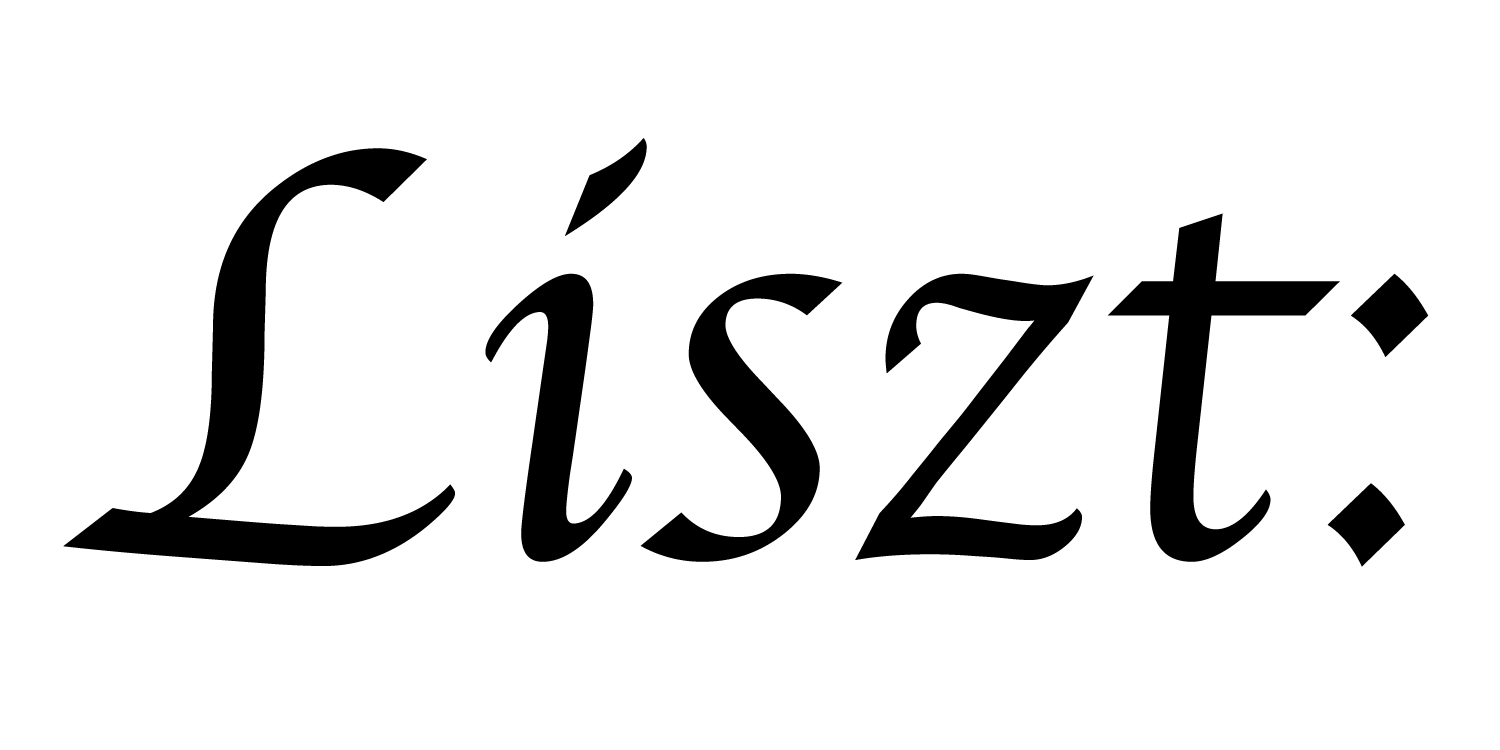# 13: Group-By and Query-Loops

In tutorial 08 (relations) we saw the most basic mechanisms for building custom geometric domain libraries: the creation of new relations and using fields of keys (key fields) to connect those relations together. Tutorials 13-17 introduce the remaining mechanisms and tricks used to model geometric domains.

In this tutorial, we’ll explore the group-by operation. To do so, we’ll use the earlier heat diffusion example from tutorial 8, except we’ll use two relations this time: one for the vertices, and one for the edges. Then, since the edges are explicitly represented, we’ll simply omit the edges that form the torus. Because we explicitly represent the edges in this way, we no longer need to assume that each vertex has exactly four neighbors in each of 4 cardinal directions.

``````import 'ebb'
local L = require 'ebblib'

local vdb   = require('ebb.lib.vdb')
``````

We start the program as usual.

``````local N = 40

local vertices  = L.NewRelation {
name = 'vertices',
size = N*N,
}
``````

We create N^2 vertices in the domain. Unlike in tutorial 08, where we encoded a toroidal topology, we’ll just omit the wrap-around edges here.

``````local edges     = L.NewRelation {
name = 'edges',
size = 4*N*(N-1),
}
``````

And we create 2*N*(N-1) horizontal edges, as well as the same number of vertical edges. These are directed edges.

``````edges:NewField('head', vertices)
edges:NewField('tail', vertices)
``````

Each edge needs to identify its head and tail vertex.

``````local tail_keys = {}
local ei        = 1
for i=0,N-1 do
for j=0,N-1 do
local vidx = i*N + j
-- left, right, bottom, top
if i > 0 then
tail_keys[ei] = vidx
ei = ei + 1
end
if i < N-1 then
tail_keys[ei] = vidx
ei = ei + 1
end
if j > 0 then
tail_keys[ei] = vidx
ei = ei + 1
end
if j < N-1 then
tail_keys[ei] = vidx
ei = ei + 1
end
end
end
``````

We compute and load the connectivity data for edges using Lua lists.

``````edges:GroupBy('tail')
``````

We group the `edges` relation by its `tail` field. This is a setup operation which tells Ebb how we plan to use the data. In particular, `GroupBy()` tells Ebb that we plan to “query” / access the edges according to which vertex their tail is.

Another way we can think of the group-by relationship is that it inverts the forward relationship established by the `tail` key-field. If we think of `tail` as a function from edges to vertices, then group-by allows us to access the pre-image of any vertex: a set of edges pointing to that vertex. We’ll see how this is used inside an Ebb function below.

``````vertices:NewField('pos',L.vec2d)

local vertex_coordinates = {}
for i=0,N-1 do
for j=0,N-1 do
vertex_coordinates[ i*N + j + 1 ] = { i, j }
end
end

``````

Since the vertices are no longer connected in a toroidal topology, we’ll go ahead and give them positions in a grid.

``````local timestep    = L.Constant(L.double, 0.45)
local conduction  = L.Constant(L.double, 1.0)
local max_diff    = L.Global(L.double, 0.0)

local function init_temperature(idx)
if idx == 0 then return 1000 else return 0 end
end

local ebb visualize ( v : vertices )
vdb.color({ 0.5 * v.t + 0.5, 0.5-v.t, 0.5-v.t })
var pos = { v.pos, v.pos, 0.0 }
vdb.point(pos)
end

local ebb measure_max_diff( e : edges )
var diff_t    = e.head.t - e.tail.t
max_diff max= L.fabs(diff_t)
end
``````

Most of the simulation code is the same as before

``````local ebb update_temperature ( v : vertices )
var sum_t = 0.0
var count = 0.0

for e in L.Where(edges.tail, v) do
count += 1
end

var avg_t  = sum_t / count
var diff_t = avg_t - v.t
v.new_t = v.t + timestep * conduction * diff_t
end
``````

However, the `update_temperature()` function now uses an unfamiliar loop. In particular, the `L.Where(edges.tail, v)` expression is called a query, and the whole loop construct is called a query loop. Read in english, it says “for each `e` in `edges` where `e.tail == v` do …”. Query loops can only be executed if the target table (`edges` here) has been prepared with a `GroupBy()` operation. Otherwise, the typechecker will throw an error.

``````for i=1,360 do
vertices:foreach(update_temperature)
vertices:Swap('t', 'new_t')

vdb.vbegin()
vdb.frame()
vertices:foreach(visualize)
vdb.vend()

if i % 10 == 0 then -- measure statistics every 10 steps
max_diff:set(0)
edges:foreach(measure_max_diff)
print( 'iteration #'..tostring(i), 'max gradient: ', max_diff:get() )
end
end
``````

The simulation loop is unchanged.

a part of the Liszt project and PSAAP II center at Stanford University